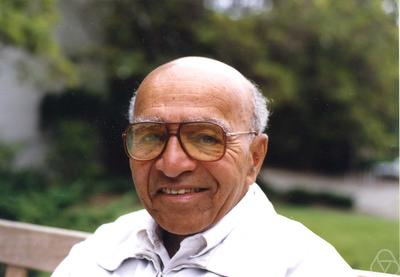# Blackwell 定理

Blackwell 定理由 David Blackwell 在 1951 年建立。值得一提的是，David Blackwell 是 UC Berkeley 第一个终身轨的黑人教授。$$! \sum_{j=1}^{m} P_{ij} \sum_{k=1}^{l} D_{jk} \cdot U_{ki} = (PDU)_{ii}$$

$$!\sum_{i=1}^{n} p_i \sum_{j=1}^{m} P_{ij} \sum_{k=1}^{l} D_{jk} \cdot U_{ki} = Trace(PDU \hat{p})$$

Blackwell 定理：考虑两个试验 $$P$$（$$n \times m$$ 维）和 $$Q$$（$$n \times m'$$ 维），以下三条等价：

1. 试验 $$P$$ 比试验 $$Q$$ 拥有更多的信息量，即对于每个 $$U$$，$$B(Q,U) \subseteq B(P,U)$$。
2. 对于每个 $$U$$ 和 $$p$$，$$F(P,U,p) \ge F(Q,U,p)$$。
3. 存在一个行随机矩阵 $$M$$（合适的维数）使得 $$P = QM$$。

“3 → 1” 和 “1 → 2” 是显然的。以下证明 “2 → 3”：

$$!Trace(G^t Q) > Trace(G^t PM)$$

（这里需要指出的是 $$n \times m'$$ 维矩阵空间上的线性泛函都是 $$Trace(G^t \cdot)$$ 的形式）

$$!Trace(PDU \hat{p}) = Trace(PDG^t) = Trace(G^t PD) < Trace(G^t Q) = Trace(QU \hat{p})$$

$$!\max_{D} Trace(PDU \hat{p}) < Trace(QU \hat{p}) \le \max_D Trace(QDU \hat{p})$$

1. Moshe Leshno and Yishay Spector, An elementary proof of Blackwell's theorem, Mathematical Social Sciences 25 (1992), 95–98.
2. Tilman Borgers, Notes on Blackwell's Comparison of Experiments, 2009.

## One thought on “Blackwell 定理”

1. lune

感谢孙老师的分享。在武大进修一年，孙老师的课是我觉得收获最大的,学到了很多精彩而有用的知识。说实话，如果孙老师在网上搞你的课程直播，我绝对会订购您的栏目的，哈哈。祝孙老师今后在学术和生活上不断完成自己的目标，取得更多成绩。

This site uses Akismet to reduce spam. Learn how your comment data is processed.